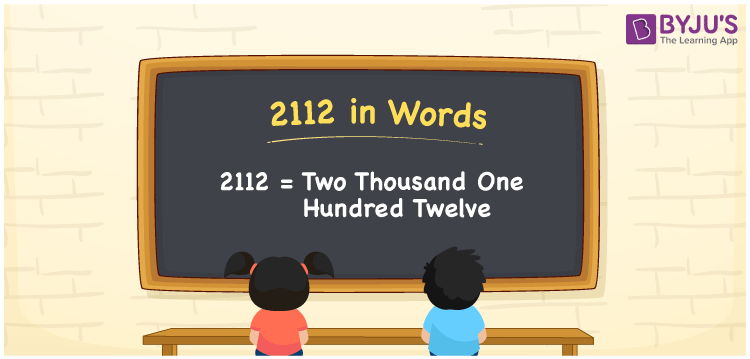# 2112 in Words

We can write 2112 in words as Two Thousand One Hundred Twelve. Suppose you have Rs. 2112 in your bank account, you can say that “I have Two Thousand One Hundred Twelve rupees in my bank account”. It is possible to convert 2112 into words with the help of a place value chart. Also, we know that 2112 is a cardinal number since it represents a specific quantity.

 2112 in words Two Thousand One Hundred Twelve Two Thousand One Hundred Twelve in Numbers 2112

## 2112 in English Words## How to Write 2112 in Words?

We can convert 2112 to words using a place value chart. This can be done as follows. The number 2112 has 4 digits, so let’s make a chart that shows the place value up to 4 digits.

 Thousands Hundreds Tens Ones 2 1 1 2

Thus, we can write the expanded form as:

2 × Thousand + 1 × Hundred + 1 × Ten + 2 × One

= 2 × 1000 + 1 × 100 + 1 × 10 + 2 × 1

= 2112

= Two Thousand One Hundred Twelve

2112 is the natural number that is succeeded by 2111 and preceded by 2113.

2112 in words – Two Thousand One Hundred Twelve

Is 2112 an odd number? – No.

Is 2112 an even number? – Yes

Is 2112 a perfect square number? – No

Is 2112 a perfect cube number? – No

Is 2112 a prime number? – No

Is 2112 a composite number? – Yes

## Frequently Asked Questions on 2112 in Words

Q1

### How to write 2112 in words?

2112 in English is written as “Two Thousand One Hundred Twelve”.
Q2

### Is 2112 a prime number?

No, the number 2112 is not a prime number. Two Thousand One Hundred Twelve is a composite number. Because 2112 has more divisors than 1 and itself.
Q3

### Write Two Thousand One Hundred Twelve in numbers.

Two Thousand One Hundred Twelve in numbers is 2112.